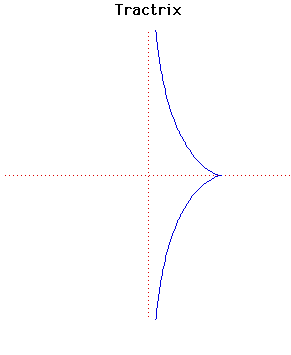# TractrixParametric Cartesian equation:
x = 1/cosh(t), y = t - tanh(t)

Click below to see one of the Associated curves.

Click THIS LINK to experiment interactively with this curve and its associated curves.

The tractrix is sometimes called a tractory or equitangential curve. It was first studied by Huygens in 1692 who gave it its name. Later Leibniz, Johann Bernoulli and others studied the curve.

The study of the tractrix started with the following problem being posed to Leibniz:

What is the path of an object dragged along a horizontal plane by a string of constant length when the end of the string not joined to the object moves along a straight line in the plane?

He solved this using the fact that the axis is an asymptote to the tractrix.

The evolute of a tractrix is a catenary. Among the properties of the tractrix are the fact that the length of a tangent from its point of contact to an asymptote is constant. The area between the tractrix and its asymptote is finite.

When a tractrix is rotated around its asymptote then a pseudosphere results. This is a surface of constant negative curvature and was used by Beltrami in 1868 in his concrete realisation of non-euclidean geometry.

You can see this
surface of revolution.

Other Web site:

JOC/EFR/BS January 1997